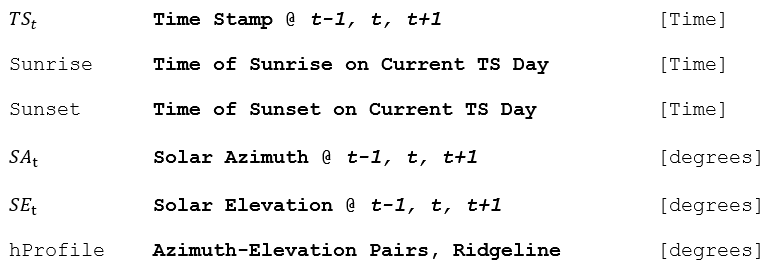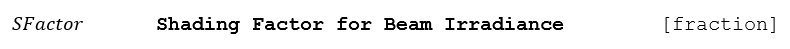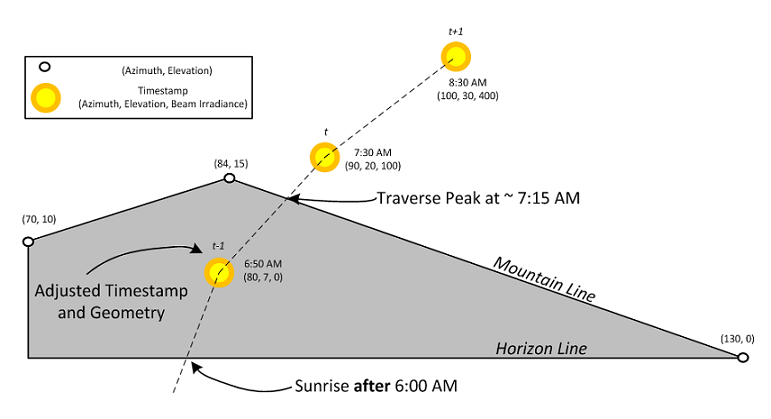## Inputs## Outputs## Algorithm Introduction

Satellite-derived irradiance data usually does not account for local topography. Horizon shading occurs when the beam component of the irradiance is occluded by a ridgeline, usually near the times of sunrise or sunset. The apparent sunrise and sunset times may be delayed and advanced, respectively, if mountains are present.

The shading effect is treated globally for the entire power plant, in other words, partial shading of a plant by distant mountains is not taken into account. This algorithm outputs a scaling value (between 0 and 1) that factors the direct beam irradiance proportional to the amount of time the sun was occluded during that simulation interval.

To estimate the amount of time the sun is blocked from far shading we use the solar geometry, the horizon profile (hProfile) and linear interpolation at 1-minute resolutions.

### The following summarizes the algorithm steps:

1.) Determine if the current timestamp could be subject to horizon shading

Using linear interpolation we can find the ridgeline elevation at any azimuth. Comparing these elevations with the sun’s elevations (at the same azimuth) over the course of the entire interval, we can determine if any horizon shading occurs.

The first step in this algorithm is to compile a list of the solar azimuth-elevation pairs (SEtSAt). If lowest solar elevation angle (has to be greater than 0) of the previous, current and next timestep is smaller than the highest elevation in the ridgeline profile then we will run the horizon shading analysis, otherwise we assume there is no shading.

2.) Estimate the time that the sun traverses the ridgeline

To determine if and when the sun traverses the ridgeline, we need to start with the timestamp that has the lowest solar elevation. The timestamps with the lowest solar elevation will always occur at the start (sunrise) or end (sunset) of a given interval. From the rotation of the earth, we know:

If 0 ≤ SAt ≤ 180 then the sun is rising and the lowest elevation is at the start of the interval, otherwise it’s at the end of the interval. For example, in the figure below, the sun is rising and the current timestamp is 7:30 AM, therefore the lowest elevation of the interval occurs at 7:00 AM. Sometime the lowest elevation of the interval is the Sunrise or Sunset time, this occurs when a sunrise or sunset event occurs during the current simulation interval.

With starting time, we can interpolate to find the solar azimuth and elevation at that time, and in turn the azimuth can be used to find the ridgeline elevation. While the ridgeline elevation is greater the solar elevation, the sun is still being obscured and we can step (interpolate) the next minute’s solar azimuth-elevation pair. Once we find the minute where the sun traverses the elevation profile, we store that time and break from the loop.### Figure 9. Example for Interpolating the Apparent Sunrise Time

3.) Estimate amount of beam irradiance that was obscured by the ridgeline

Now that we know the solar transit time above the ridgeline time, we need to calculate how much beam irradiance was blocked by the ridgeline. Consider the configuration diagramed in figure 9 above. Our current middle-of-irradiance interval timestamp is 7:30 AM and the transit time is 7:15 AM. If we found the transit time to be before 7:00 AM then we would have no shading to calculate, but in this case we know that the sun was obscured for 15 minutes of the current interval. We then estimate the amount of beam shading by finding what fraction of the interval was occluded, in this case 15 min / 60 min or 25%. This corresponds to a beam shading factor or SFactor of 0.72.

There are shortcomings of this algorithm, namely that it tends to overpredict beam shading because it treats the irradiance over every minute of interval as equal. In reality, the irradiance varies throughout the hour and therefor the shading factor should reflect this. This could be achieved in the future by interpolating the irradiances at t-1,t and t+1 to come up with a more accurate shading factor value.

Additionally, the algorithm could be improved by reducing the diffuse ground and diffuse sky irradiances but both of these changes need to be motivated by measured data before they can be implemented.